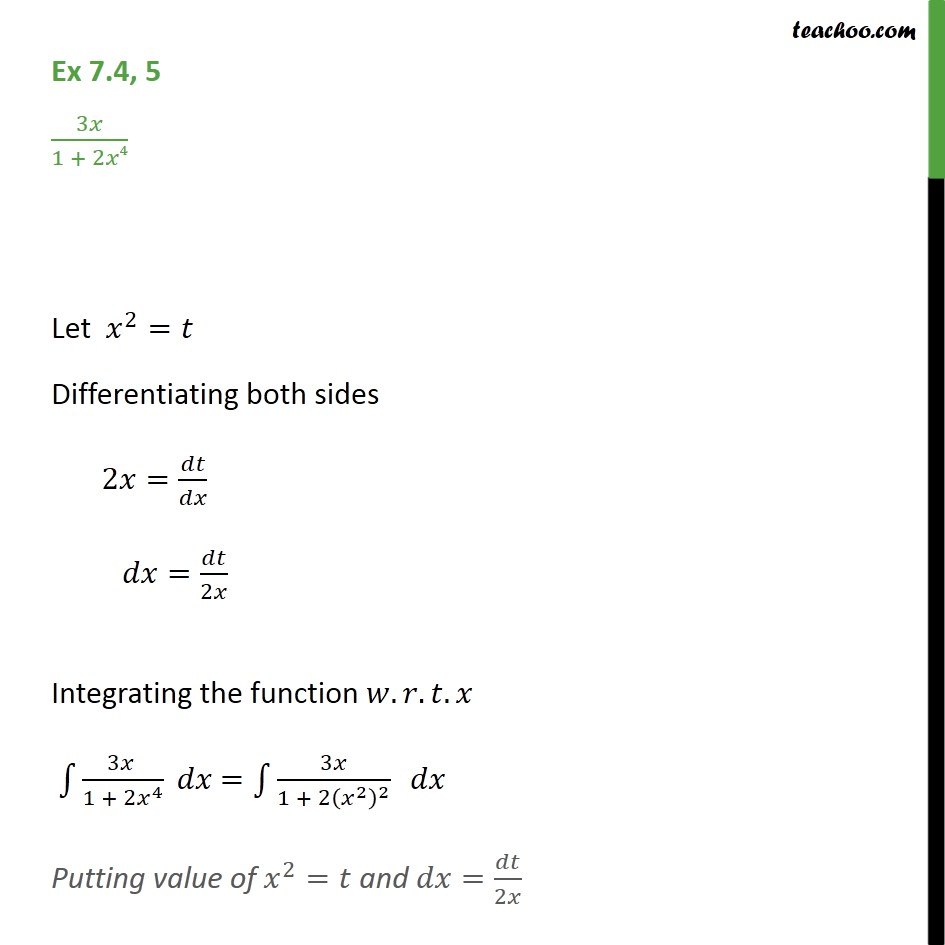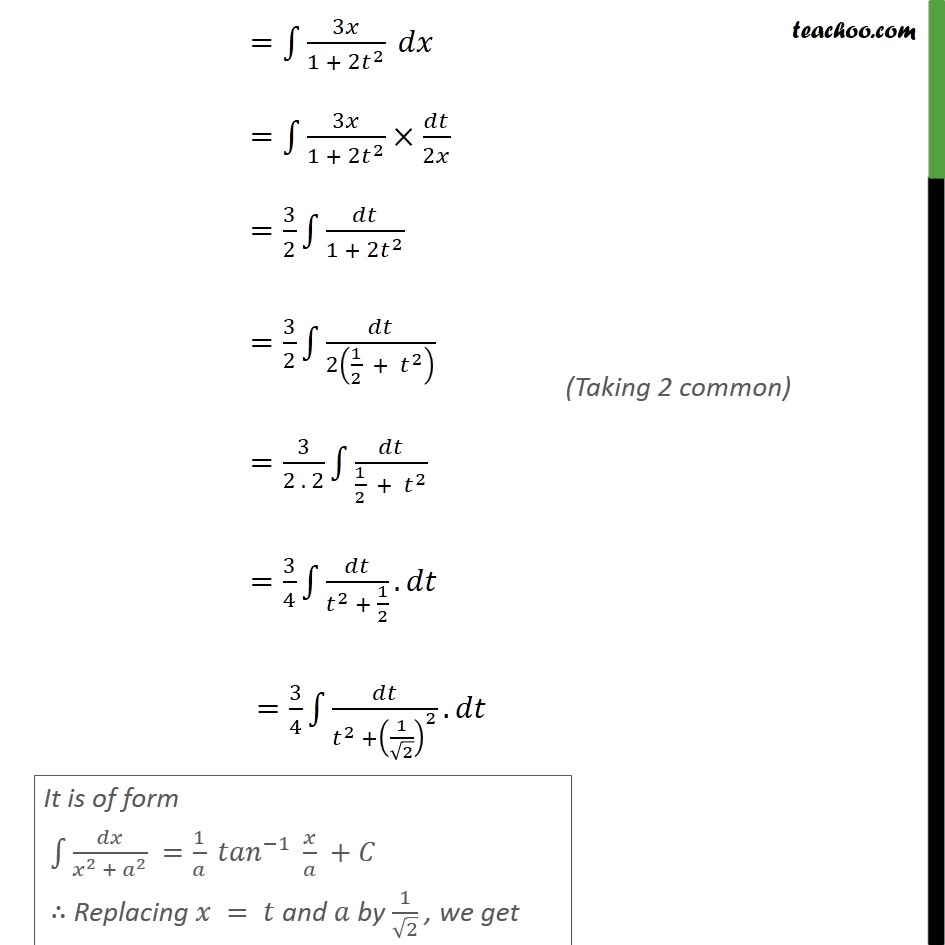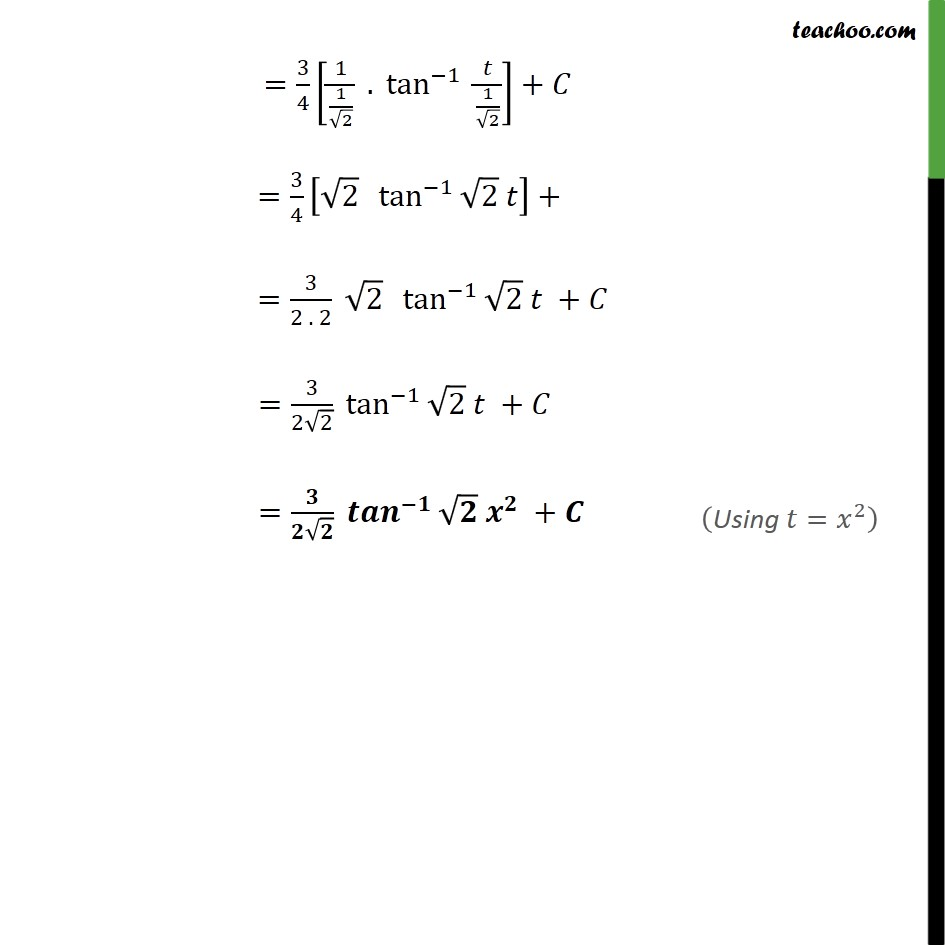Ex 7.4

Chapter 7 Class 12 Integrals
Serial order wiseLearn in your speed, with individual attention - Teachoo Maths 1-on-1 Class

### Transcript

Ex 7.4, 5 3𝑥/(1 + 2𝑥4) Let 𝑥^2=𝑡 Differentiating both sides 2𝑥=𝑑𝑡/𝑑𝑥 𝑑𝑥=𝑑𝑡/2𝑥 Integrating the function 𝑤.𝑟.𝑡.𝑥 ∫1▒(3𝑥 )/(1 + 2𝑥^4 ) 𝑑𝑥 =∫1▒3𝑥/(1 + 2(𝑥^2 )^2 ) 𝑑𝑥 Putting value of 𝑥^2=𝑡 and 𝑑𝑥=𝑑𝑡/2𝑥 =∫1▒3𝑥/(1 + 2𝑡^2 ) 𝑑𝑥 =∫1▒3𝑥/(1 + 2𝑡^2 )×𝑑𝑡/2𝑥 =3/2 ∫1▒𝑑𝑡/(1 + 2𝑡^2 ) =3/2 ∫1▒𝑑𝑡/2(1/2 + 𝑡^2 ) =3/(2 . 2) ∫1▒𝑑𝑡/(1/2 + 𝑡^2 ) =3/4 ∫1▒𝑑𝑡/(𝑡^2 + 1/2). 𝑑𝑡 =3/4 ∫1▒𝑑𝑡/(𝑡^2 +(1/√2)^2 ). 𝑑𝑡